# DAV Class 3 Maths Chapter 7 Brain Teasers Solutions

The DAV Class 3 Maths Solutions and DAV Class 3 Maths Chapter 7 Brain Teasers Solutions of Money offer comprehensive answers to textbook questions.

## DAV Class 3 Maths Ch 7 Brain Teasers Solutions

Question 1.

(a) Rahul has ₹ 120. He wants to buy a toy robot that costs ₹ 180. How much more money does he need?
(i) ₹ 150
(ii) ₹ 50
(iii) ₹ 60
(iv) ₹ 90
Solution:
(iii) ₹ 60

Cost of the toy robot = ₹ 180
Amount Rahul had = ₹ 120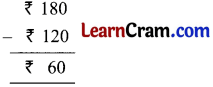Amount needed = ₹ 60

(b) How many 50 paise coins are there in a 10 rupee note?
(i) 25 coins
(ii) 35 coins
(iii) 30 coins
(iv) 20 coins
Solution:
(iv) 20 coins
In 1 rupee = two 50 paise coins
In 10 rupees = (2 × 10) 50 Paise coins
In 10 rupees = 20 50 Paise coins(c) 80 rupees is equal to
(i) 800 paise
(ii) 8000 paise
(iii) 0.80 paise
(iv) 80 paise
Solution:
(ii) 8000 paise
1 rupee = 100 paise
80 rupees = 100 × 80 paise
= 8000 paise

(d) Aman had ₹ 90. He purchased a pen for ₹ 55. How much money was left with him?
(i) ₹ 50
(ii) ₹ 45
(iii) ₹ 35
(iv) ₹ 40
Solution:
(iii) ₹ 35
Aman had money = ₹ 90
Cost of pen = ₹ 55Money left = ₹ 35

(e) Which of the following coins does not exist in Indian currency?
(i) ₹ 2
(ii) ₹ 5
(iii) ₹ 1
(iv) ₹ 3
Solution:
(iv) ₹ 3Question 2.
Write in figures.

(a) 63 rupees 50 paise
Solution:
63 rupees 50 paise = ₹ 63.50

(b) 7 paise
Solution:
7 paise = ₹ 0.07

(c) 3 rupees 15 paise
Solution:
3 rupees 15 paise = ₹ 3.15

(d) 28 paise
Solution:
28 paise = ₹ 0.28Question 3.
Write in words.

(a) ₹ 31.55
Solution:
Thirty one rupees and fifty five paise.

(b) ₹ 0.08
Solution:
Eight paise

(c) ₹ 5.15
Solution:
Five rupees and fifteen paise

(d) ₹ 70.07
Solution:
Seventy rupees and seven paiseQuestion 4.
Add ₹ 36.53, ₹ 0.08 and ₹ 15.58
Solution:Question 5.
Subtract 45 paise from 2 rupees.
Solution:Question 6.
From a book fair, Arun purchased 3 books costing ₹ 15.50, ₹ 26.50 and ₹ 5.50. If he pays ₹ 50, how much balance will he get back?
Solution:Total cost of books = ₹ 47.50
Amount left = ₹ 50.00 – ₹ 47.50 = ₹ 2. 50He will get back = ₹ 2.50Question 7.
Peter purchased the following items from the Super Bazar.
Toilet soap = ₹ 24.00
Shaving Cream = ₹ 52.50
Toothpaste = ₹ 47.50
Hair Oil = ₹ 76.00
Cold Cream = ₹ 92.50
Prepare a cash memo amount paid by him. and find the total amount paid by him
Solution:The total amount paid by him is ₹ 292.50.

Question 8.
Look at the following cash memo and find out the mistakes and correct them.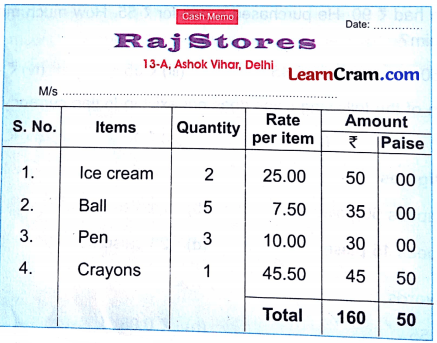Solution:
Correct cash memo is the following:Question 1.
Convert the following into paise.

(a) ₹ 68
Solution:
₹ 68 = 68 × 100 p
= 6800 p

(b) ₹ 8.50
Solution:
₹ 8.50 = (8 × 100 + 50) p
= (800 + 50) P
= 850 p

(c) ₹ 87.75
Solution:
₹ 87.75 = 8775 PQuestion 2.
Convert the following into rupees.

(a) 45325 paise
Solution:
45325 P = 45325 ÷ 100
= ₹ 453.25
= 453 rupees 25 paise

(b) 3225 paise
Solution:
3225 P = 3225 ÷ 100
= ₹ 32.25
= 32 rupees 25 paise

(c) 8 paise
Solution:
8 Paise = ₹ 0.08Question 3.

(a)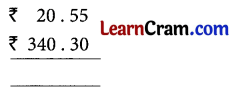Solution:
₹ 360.85

(b)Solution:
₹630.25

(c)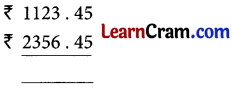Solution:
₹ 3479.90

(d)Solution:
₹ 108.20Question 4.

(a)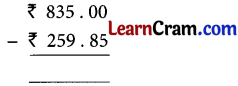Solution:
₹ 575.15

(b)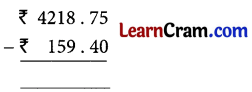Solution:
₹ 4059.35

(c)Solution:
₹ 6250.80

(d)Solution:
₹ 111.30Question 5.
Find the mistakes in following cash memo and correct them.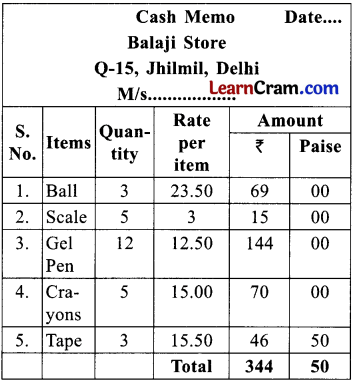Solution: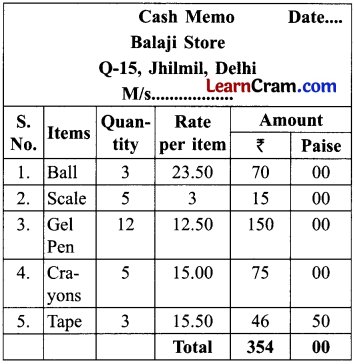Question 6.
Multiplication of money

(a) ₹ 101.00 × 4
Solution:
₹ 404

(b) ₹ 72.50 × 3
Solution:
₹ 217.50

(c) ₹ 15.25 × 2
Solution:
₹ 30.50

(d) ₹ 0.75 × 5
Solution:
₹ 3.75Question 7.
Farukh bought a bag for ₹ 450 and cap for ₹ 300.25. He gave a ₹ 1000 note to the shopkeeper. How much money did the shopkeeper return?
Solution:
Cost of the bag = ₹ 450.00
Cost of cap = ₹ 300.25Total cost = ₹ 750.25

Amount Farukh gave to shopkeeper = ₹ 1000.00
Money spent = ₹ 750.25Money left = ₹ 249 . 75
The shopkeeper returned ₹ 249.75.